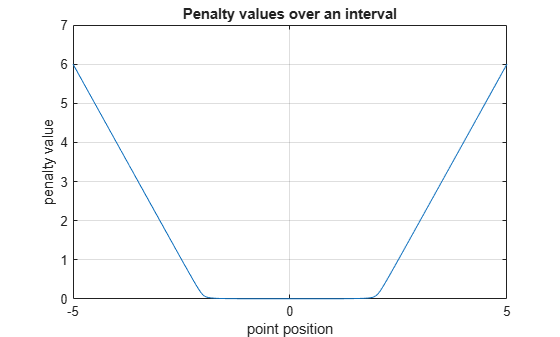hyperbolicPenalty

Hyperbolic penalty value for a point with respect to a bounded region

Description

example

p = hyperbolicPenalty(x,xmin,xmax) calculates the nonnegative (hyperbolic) penalty vector p for the point x with respect to the region bounded by xmin and xmax. p has the same dimension as x. This syntax uses the default values of 1 and 0.1 for the lambda and tau parameters of the hyperbolic function, respectively.

example

p = hyperbolicPenalty(___,lambda,tau) specifies both the lambda and tau parameters of the hyperbolic function. If lambda is an empty matrix its default value is used. Likewise if tau is an empty matrix or it is omitted, its default value is used instead.

Examples

collapse all

This example shows how to use the hyperbolicPenalty function to calculate the hyperbolic penalty for a given point with respect to a bounded region.

Calculate the penalty value for the point 0.1 within the interval [-2,2], using default values for the lambda and tau parameters.

hyperbolicPenalty(0.1,-2,2)
ans = 0.0050

Calculate the penalty value for the point 4 outside the interval [-2,2].

hyperbolicPenalty(4,-2,2)
ans = 4.0033

Calculate the penalty value for the point 0.1 within the interval [-2,2], using a lambda parameter of 5.

hyperbolicPenalty(0.1,-2,2,5)
ans = 0.0010

Calculate the penalty value for the point 4 outside the interval [-2,2], using a lambda parameter of 5.

hyperbolicPenalty(4,-2,2,5)
ans = 20.0007

Calculate the penalty value for the point 4 outside the interval [-2,2], using a tau parameter of 0.5.

hyperbolicPenalty(4,-2,2,5,0.5)
ans = 20.0167

Calculate the penalty value for the point [-2,0,4] with respect to the box defined by the intervals [0,1], [-1,1], and [-2,2] along the x, y, and z dimensions, respectively, using the default value for lambda and a tau parameter of 0.

hyperbolicPenalty([-2 0 4],[0 -1 -2],[1 1 2],1,0)
ans = 3×1

4
0
4

Visualize Penalty Values for an Interval

Create a vector of 1001 equidistant points distributed between -5 and 5.

x = -5:0.01:5;

Calculate penalties for all the points in the vector, using default values for the lambda and tau parameters.

p = hyperbolicPenalty(x,-2,2);

Plot the points and add grid, labels and title.

plot(x,p)
grid
xlabel("point position");
ylabel("penalty value");
title("Penalty values over an interval");Input Arguments

collapse all

Point for which the penalty is calculated, specified as a numeric scalar, vector or matrix.

Example: [0.5; 1.6]

Lower bounds for x, specified as a numeric scalar, vector or matrix. To use the same minimum value for all elements in x specify xmin as a scalar.

Example: -1

Upper bounds for x, specified as a numeric scalar, vector or matrix. To use the same maximum value for all elements in x specify xmax as a scalar.

Example: 2

Lambda parameter of the hyperbolic function, specified as a scalar.

Example: 3

Tau parameter of the hyperbolic function, specified as a scalar.

Example: 0.3

Output Arguments

collapse all

Penalty value, returned as a vector of nonnegative elements. Each element pi depends on the position of xi with respect to the interval specified by xmini and xmaxi. The hyperbolic penalty function returns the value:

$p\left(x\right)=-\lambda \left(x-{x}_{\mathrm{min}}\right)+\sqrt{{\lambda }^{2}{\left(x-{x}_{\mathrm{min}}\right)}^{2}+{\tau }^{2}}-\lambda \left({x}_{\mathrm{max}}-x\right)+\sqrt{{\lambda }^{2}{\left({x}_{\mathrm{max}}-x\right)}^{2}+{\tau }^{2}}$

Here, λ is the argument lambda, and τ is the argument tau. Note that for positive values of τ the returned penalty value is always positive, because on the right side of the equation the magnitude of the second term is always greater than that of the first, and the magnitude of the fourth term is always greater than that of the third. If τ is zero, then the returned penalty is zero inside the interval defined by the bounds, and it grows linearly with x outside this interval. If x is multidimensional, then the calculation is applied independently on each dimension. Penalty functions are typically used to generate negative rewards when constraints are violated, such as in generateRewardFunction.